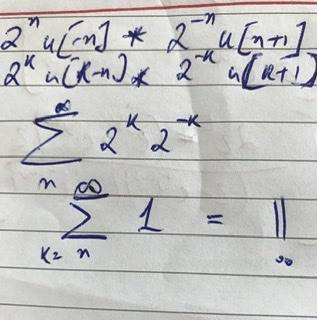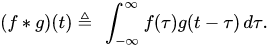# Convolution Sum

Summary:
What all does folding a signal entail?
Please see below my attempt to perform the convolution operation on two discrete-time signals as part of my Digital Signal Processing class.I suspect my folding operation, i.e. flipping one signal about k=0, might be the cause.

Ostensibly the answer of the convolution sum evaluated at n=-2 should be 4/3.

Would appreciate if you can point out my error.

Thank you and Namaste

## Answers and Replies

BvU
Science Advisor
Homework Helper
two discrete-time signals as part of my Digital Signal Processing class.
Care to share the complete problem statement with us ? Not just a snippet with rather random scribbles and no indication of what is wrapping and what is not, no logic, no punctuation, ... ?

Care to share the complete problem statement with us ?

Thank you for responding.
Hope today has been good one.

My apologies if the problem statement wasn’t ostensible. The two signals are in the first line; flipping, which is one of the steps of discrete-time convolution, occurs in the second.

Problem Statement: Perform convolution sum on the two signals shown in the first line.

Namaste.

BvU
Science Advisor
Homework Helper
The two signals are in the first line; flipping, which is one of the steps of discrete-time convolution, occurs in the second.
Good.

So line 1 has two signals. The first being sample(##n##) ##= 2^n [u(-n)]## and the second being ##2^{-n} [u(n+1)]## without further specification of ##u(n)## ?

How can 'flipping' (?) lead to line 2 ?

What is the relation between the third line and the preceding ones ?

And: how do I distinguish beween u, n and k in your handwriting ?

Then: I'm familiar with convolutions likeso I suppose your R is the equivalent of ##\tau## ?

## ##

Note that you are doing a discrete-time convolution. Try plotting (e.g., a stem plot) just the two step functions (i.e., u[n]). Look at them visually. One is reversed and the other is time shifted. Then decide which one you will reverse (i.e., flip) for the convolution operation. Do it, and look again visually. That will tell you the summation range that is relevant. Plug everything into the discrete-time convolution equation and evaluate. It is important to realize that step functions are used to limit the summation range for discrete convolutions and transforms. Have fun!

Edit - you will also need some useful expressions. See slide 2 of HERE

Last edited:
•berkeman